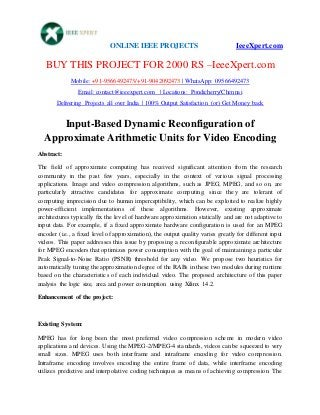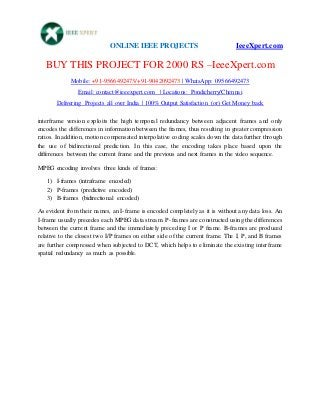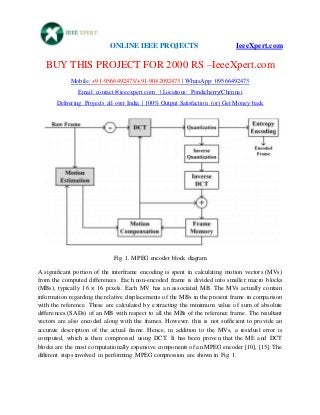Successfully reported this slideshow.

# Input based dynamic reconfiguration of approximate arithmetic units for video encoding×

# Input based dynamic reconfiguration of approximate arithmetic units for video encoding

Input based dynamic reconfiguration of approximate arithmetic units for video encoding Input based dynamic reconfiguration of approximate arithmetic units for video encoding Input based dynamic reconfiguration of approximate arithmetic units for video encoding Input based dynamic reconfiguration of approximate arithmetic units for video encoding Input based dynamic reconfiguration of approximate arithmetic units for video encoding Input based dynamic reconfiguration of approximate arithmetic units for video encoding Input based dynamic reconfiguration of approximate arithmetic units for video encoding

Input based dynamic reconfiguration of approximate arithmetic units for video encoding Input based dynamic reconfiguration of approximate arithmetic units for video encoding Input based dynamic reconfiguration of approximate arithmetic units for video encoding Input based dynamic reconfiguration of approximate arithmetic units for video encoding Input based dynamic reconfiguration of approximate arithmetic units for video encoding Input based dynamic reconfiguration of approximate arithmetic units for video encoding Input based dynamic reconfiguration of approximate arithmetic units for video encoding